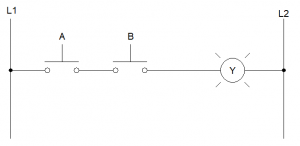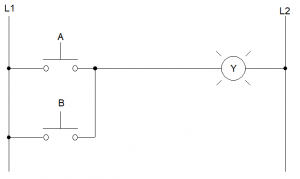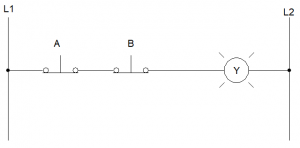# 2.3: Introduction to Logic

$$\newcommand{\vecs}{\overset { \rightharpoonup} {\mathbf{#1}} }$$ $$\newcommand{\vecd}{\overset{-\!-\!\rightharpoonup}{\vphantom{a}\smash {#1}}}$$$$\newcommand{\id}{\mathrm{id}}$$ $$\newcommand{\Span}{\mathrm{span}}$$ $$\newcommand{\kernel}{\mathrm{null}\,}$$ $$\newcommand{\range}{\mathrm{range}\,}$$ $$\newcommand{\RealPart}{\mathrm{Re}}$$ $$\newcommand{\ImaginaryPart}{\mathrm{Im}}$$ $$\newcommand{\Argument}{\mathrm{Arg}}$$ $$\newcommand{\norm}{\| #1 \|}$$ $$\newcommand{\inner}{\langle #1, #2 \rangle}$$ $$\newcommand{\Span}{\mathrm{span}}$$ $$\newcommand{\id}{\mathrm{id}}$$ $$\newcommand{\Span}{\mathrm{span}}$$ $$\newcommand{\kernel}{\mathrm{null}\,}$$ $$\newcommand{\range}{\mathrm{range}\,}$$ $$\newcommand{\RealPart}{\mathrm{Re}}$$ $$\newcommand{\ImaginaryPart}{\mathrm{Im}}$$ $$\newcommand{\Argument}{\mathrm{Arg}}$$ $$\newcommand{\norm}{\| #1 \|}$$ $$\newcommand{\inner}{\langle #1, #2 \rangle}$$ $$\newcommand{\Span}{\mathrm{span}}$$$$\newcommand{\AA}{\unicode[.8,0]{x212B}}$$

## Logic in Electronics

While this lesson is a brief introduction to logic, understand that almost no part of our modern society can function without the touch of digital electronics. Everything from our TVs to phones, cars to gaming consoles, and computers to even the cash registers at the local grocery store would not be possible without these electronic devices. In this lesson, you’ll learn how to hard-wire these types of logical functions. Later, when you learn to use Programmable Logic Controllers (PLCs), you’ll also learn about the small electronic devices that perform the following functions.

#### ANDLogical AND

In words, the logical AND sounds something like this, “If A AND B are pressed, then Y is energized.”

Considering A and B as our inputs, we can determine when Y (our output) is energized. Folks sometimes use a truth table to help analyze these functions. We use binary (using only the numerals 0 and 1) to help represent the state of our circuit. Binary can be used any time something only has two states (true/false, high/low, yes/no, open/closed, etc.). If “0” is the normal state, and “1” denotes an activated or energized state, let’s look a truth table for the AND function.

 AND A B Y 0 0 0 0 1 0 1 0 0 1 1 1

The table is read row-by-row. In the first case, neither A or B is pressed, and Y is not activate. In the second row, A is not pressed, B is pressed, and Y is not energized. Notice that Y is only energized when both A and B are pressed. In any other case, lamp Y is not lit.

#### ORLogical OR

In words, the logical OR description might be, “If button A OR B are pressed, then Y is energized.”

The truth table for OR logic looks like the following. Notice that in this case, the only time lamp Y is not lit is in the first row when neither button is pressed. In all other cases, Y is energized.

 OR A B Y 0 0 0 0 1 1 1 0 1 1 1 1

#### NOTLogical NOT

Not is the simplest logical operation. It simply takes the input and inverts it. In our case, if button A is NOT pressed, then Y is energized (you could also say that if A is pressed, then Y is NOT energized). The truth table is shorter, since there’s only one input, and it looks like this:

 OR A Y 0 1 1 0

#### NORLogical NOR

In the case of NOR, Y is energized as long as neither A NOR B is pushed. Notice that the truth table is an exact inverse of OR. In the case of OR, Y was energized if either button was pressed. With NOR, Y is de-energized if either button is pushed.

 NOR A B Y 0 0 1 0 1 0 1 0 0 1 1 0

#### NANDLogical NAND

With NAND, if both A and B are not pressed, then Y is energized. Notice the wording NOT AND. Looking at the truth table might confirm your suspicions. The output column for NAND is an inverse of the AND truth table.

 NAND A B Y 0 0 1 0 1 1 1 0 1 1 1 0

#### XORLogical XOR

XOR (pronounced “ex-or” or “exclusive or”) allows us to energize Y if either A or B is pressed, but not if both are pressed. So, it’s function is like OR logic, but the load is not energized if both buttons are pressed.

This page titled 2.3: Introduction to Logic is shared under a CC BY-SA 4.0 license and was authored, remixed, and/or curated by Ken Dickson-Self (OpenOregon) via source content that was edited to the style and standards of the LibreTexts platform; a detailed edit history is available upon request.